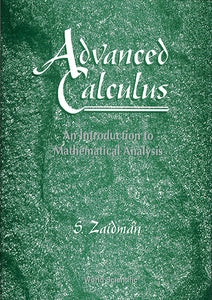# Advanced Calculus, An Introduction To Mathematical Analysis

Samuel Zaidman

Format: Print Book

ISBN: 9789810227043

• \$71.13
Unit price per
Tax included.

This book is an introduction to mathematical analysis (i.e real analysis) at a fairly elementary level. A great (unusual) emphasis is given to the construction of rational and then of real numbers, using the method of equivalence classes and of Cauchy sequences. The text includes the usual presentation of: sequences of real numbers, infinite numerical series, continuous functions, derivatives and Riemann-Darboux integration. There are also two “special” sections: on convex functions and on metric spaces, as well as an elementary appendix on Logic, Set Theory and Functions. We insist on a rigorous presentation throughout in the framework of the classical, standard, analysis.

Format: Hardcover
No of Pages: 180
Imprint: World Scientific
Publication date: 19970807